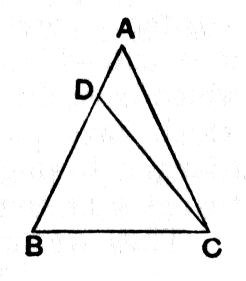#Elements: Book I, Proposition 6

Math Lair Home > Source Material > Elements > Book I, Proposition 6

The following is as given in Sir Thomas L. Heath's translation, which can be found in the book The Thirteen Books of The Elements, Vol. 1.

Elements on the Math Lair
Book IBook IIBook IX
Definitions, Postulates, and Common NotionsDefinitionsProposition 20
Proposition 1, Proposition 3, Proposition 14Proposition 36
Proposition 5, Proposition 6,
Proposition 29, Proposition 47

## Proposition 6.

If in a triangle two angles be equal to one another, the sides which subtend the equal angles will also be equal to one another.

Let ABC be a triangle having the angle ABC equal to the angle ACB;I say that the side AB is also equal to the side AC.

For, if AB is unequal to AC, one of them is greater.

Let AB be greater; and from AB the greater let DB be cut off equal to AC the less;

let DC be joined.

Then, since DB is equal to AC,

and BC is common,

the two sides DB, BC are equal to the two sides AC, CB respectively;

and the angle DBC is equal to the angle ACB;
therefore the base DC is equal to the base AB,

and the triangle DBC will be equal to the triangle ACB,

the less to the greater;

which is absurd.

Therefore AB is not unequal to AC;

it is therefore equal to it.

Therefore etc.

Q.E.D.